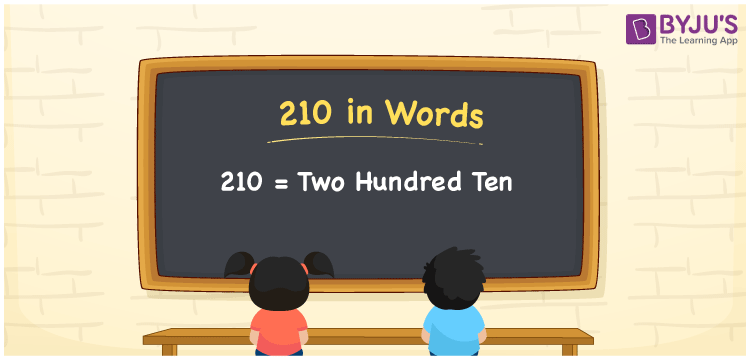# 210 in words

210 in words is written as Two Hundred and Ten. 210 represents the count or value. The article on Counting Numbers can give you an idea about count or counting. The number 210 is used in expressions that relate to money, days, distance, length, weight and so on. Let us consider an example for 210. “I believed there were Two Hundred and Ten bones in a human body, until I attended a session on Human Anatomy.” Another example, “This palace is Two Hundred and Ten years old.”

 210 in words Two Hundred and Ten Two Hundred and Ten in Numbers 210

## 210 in English Words## How to Write 210 in Words?

We can convert 210 to words using a place value chart. The number 210 has 3 digits, so let’s make a chart that shows the place value up to 3 digits.

 Hundreds Tens Ones 2 1 0

Thus, we can write the expanded form as:

2 × Hundred + 1 × Ten + 0 × One

= 2 × 100 + 1 × 10 + 0 × 1

= 210

= Two Hundred and Ten.

210 is the natural number that is succeeded by 209 and preceded by 211.

210 in words – Two Hundred and Ten.

Is 210 an odd number? – No.

Is 210 an even number? – Yes.

Is 210 a perfect square number? – No.

Is 210 a perfect cube number? – No.

Is 210 a prime number? – No.

Is 210 a composite number? – Yes.

## Solved Example

1. Write the number 210 in expanded form

Solution: 2 × 100 + 1 × 10 + 0 × 1

We can write 210 = 200 + 10 + 0

= 2 × 100 + 1 × 10 + 0 × 1

## Frequently Asked Questions on 210 in words

Q1

### How to write 210 in words?

210 in words is written as Two hundred and Ten.
Q2

### Is 210 divisible by 2?

Yes. 210 is divisible by 2.
Q3

### Is 210 a perfect square number?

No. 210 is not a perfect square number.